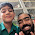Jun 9, 2011

Coin Chain Reaction

Source: Asked to me by Prateek Srivastava (IITB CSE Alumnus 2010 and Yahoo! Software Engineer) (also asked in a quant firm placement test)

Problem:
We have an unlimited number of dice at our disposal. Let's roll the die. If the outcome is 1, 2, or 3, we stop; otherwise, if it is 4, 5, or 6, a corresponding number of dice are rolled. For example, if the first roll gives 5, then we roll 5 dice, and so on. This procedure continues for every rolled dice whose outcome is 4, 5, or 6. Let N denote the N-th round of rolls. What is the total expected value at the end of the N-th round of rolls?

Update (17 July 2011):
Solution posted by Unknown in comments. There is a slight ambiguity in the problem statement, so please do not waste a lot of time. Thanks.

1.E[N+1] denotes expected number of dice at N+1 stage.
E[N+1]= E[N]*E
This is because, the process can be modeled as each die at the N stage starting a new process similar to the parent process.
Hence, the expected number of dice for each die in stage N is E, where E denotes the expected number of dice after the first throw.
E = (4+5+6)/6 = 2.5
Hence, the expected number of dice at the N+1th round of rolls will be (2.5*E[N]). This implies that E[N] = 2.5^N
Note that this is a sort-of intuitive argument (the expected number of dice could be non integral).
To make the argument concrete, consider Xn to be the random variable representing the number of dice at Nth stage.
Now, Xn+1 = Random variable representing number of dice at n+1 th stage.
Xn+1 = summation(X1)[Xn times]
An important (but reasonable) assumption is that Xn and X1 are independent.
Hence, E(Xn+1) = E(Xn)*E(X1)

Hence, expected number of dice after the Nth round of rolls is (E(X1))^N = (2.5)^N

2.I think total expected number at the end of Nth round of rolls is 2*((2.5)^N-1)/3.
the expected no of dice rolled in nth round is (2.5)^(N-1) if we consider roll of 1st dice as 1st round.

3.I think total expected number at the end of Nth round of rolls is 2*((2.5)^N-1)/3.
the expected no of dice rolled in nth round is (2.5)^(N-1) if we consider roll of 1st dice as 1st round

4.it shud be (3.5)* (2.5)^n-1

5.This comment has been removed by the author.

6.is it (3.5)*(2.5)^N-1 ?..

7.@Everyone..
We all see the 2.5^(N-1) part..

I think it should be 3.5*() but its not correct somehow..

@Unknown, How did you come up with 2/3*()?

Thanks.

8.If I have read the problem correctly the total number FROM the i-th round itself should be 3.5*(2.5)^(i-1) as everyone has posted. This then gives us the total AFTER the n-th round should be \sum_{i=1}^n 3.5*(2.5^(i-1)) which equals 3.5*(1-2.5^n)/(1-2.5)=7/3*(2.5^n-1).

Note: For n=1 we get 7/3*(2.5^1-1)=7/2 and 1/6*(1+2+3+4+5+6)=7/2! Also, for n=2 we get 7/3*(2.5^2-1)=49/4 and 1/6*[1+2+3+(4+4*7/2)+(5+5*7/2)+(6+6*7/2]=49/4!

Let me know what you think, James.

9.I guess question have asked the total expected no. of rolls of dices at the end of the N-th round. So I just added all the expected no. dices rolled from round 1 to round N
1+2.5^1+2.5^2.....+2.5^N-1=
2*(((2.5)^N)-1)/3
I don't know if my view was correct or not.

10.@Unknown..
The question is a multiple choice question from the placement test. The option 3.5*() was not there. So, I guess your solution is correct.

@Others.. sorry for the ambiguity.

Thanks.

11.Hi...
Shouldn't the constant in unknown's solution be equal to 3. If {1,2,3} comes after the first roll then the number of dice remains 1 whereas it becomes {4,5,6} if the respective figure comes on the roll. Ergo, E(1) = (1+1+1+4+5+6)/6 = 3.
Please correct me if I am wrong.
Thanks!!! :)

Fraction Brainteaser

Source: Sent to me by Gaurav Sinha Problem: Siddhant writes a Maths test and correctly answers 5 out of 6 Arithmetic questions and 20...